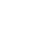# Power Factor Correction Calculator

2
14148This is the power factor correction calculator. With the help of this calculator you can find the value of kVAr required to compensate the power factor. Compensation of KVar is must if you want to correct power factor in your plant or factory.

The calculator is very simple to use. Just enter the kW(real power). Enter the existing power factor and target power factor. That is all you need. The result displayed is in require KVar.

 .specialclass lblTest { margin-left: 200px; }

There are other methods also to calculate KVar, with the help of variables like capacitance, frequency and current of capacitor. But personally speaking, the above one is the simplest one.

I would show you the formula used for calculation.Where,

Qc = reactive power needed

P = real or active power

φ1= actual angle of cosφ actual

φ2= target angle of cosφ target

You are not suppose to convert cosφ to tanφ. The calculator would do it automatically once you enter the values of actual cosφ and target cosφ. However, if you still have desire to know how it is done, this is the formula.Now let us take a look on one example.

Example
Consider a system having 1000 kW as active power having existing power factor cosφ1 as 0.65 and target power factor cosφ2 is 0.96. The required KVar is 880 KVar.

Feel free to ask me any question in case if you still have doubts.

1.niket
2.Anilkumar